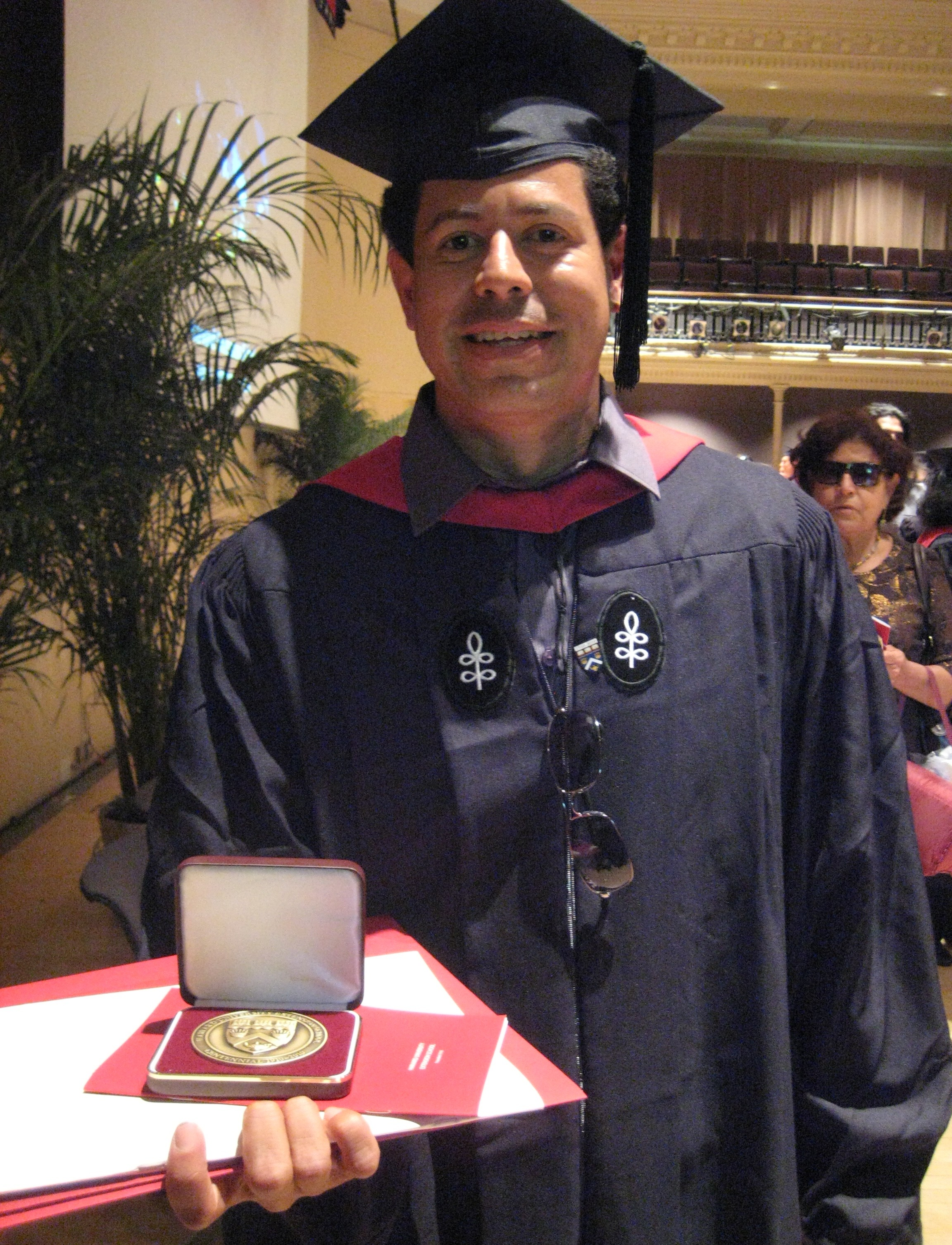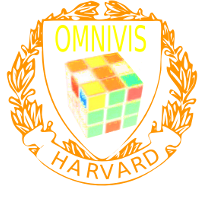OMNIVIS project 2009
Jose Ramirez theses on Structure from Motion
OMNIVIS: Omni-directional Vision
Office: SciCtr 434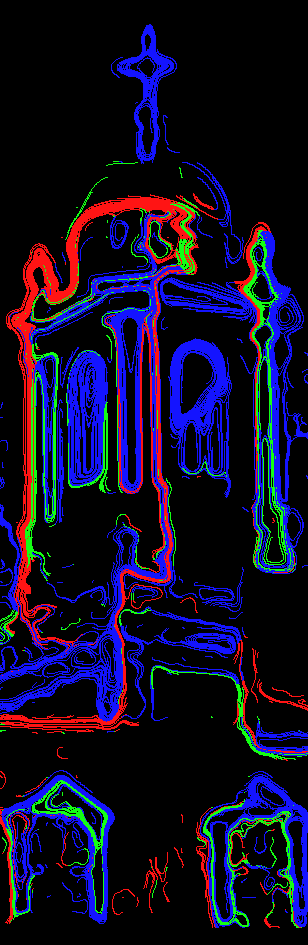The illustrations were "painted" automatically using the Hamiltonian flow x' = J nabla H(x), where H is a red green or blue function interpreted as an energy. The paths follow the level curves. The "pens" started at points with the corresponding color had large curvature or gradient. Absolutely no after processing has been done. The flow paints the relevant lines automatically. This Harvard extension school thesis project of Jose Ramirez builds on work done in the summer of 2007, where the mathematics of the structure from motion problem in general and for affine and omnidirectional cameras was studied. The reconstruction with synthetic pictures worked beautifully, if the correspondence of points in different frames is known. The engineering thesis project aims to reconstruct in Povray generated scenes in a semi synthetic approach, where the computer films the scene and where the reconstruction is done from a movie. This still needs more work, but the underlying low level image and movie analysis tools are now solidly built from scratch in C. Not a single line of code was borrowed from previous work done in the field. Everything is done consistently in color space and not with gray level pictures. In order to do the reconstruction, the correspondence problem has to be solved. This requires low level programming in order to be able to analyze the structure of a movie. Using literature as background, the reconstruction program is done from scratch: various geometric quantities like gradient, curvature of the red, green and blue functions of a picture are computed to find interesting points. One of the difficulties is the huge amount of parameters which can be varied: smoothing level, patch sizes, grid sizes, discretization settings, thresholds. The reconstruction will then be done with Mathematica using the already developed mathematics. The subject is an application of multivariable calculus, linear algebra and differential equations: even so things are discrete, the mathematics of smooth functions is suited in computer vision: a picture is given by functions r(x,y),g(x,y),b(x,y) representing the red, green and blue colors. Their gradients, Hessians, level curves, level curvatures are essential to find features in the frames. In a movie, these functions change in time and are given by functions r(x,y,t),g(x,y,t),b(x,y,t) of three variables. Tracking points means to find curves (xk(t),yk(t)) in the plane such that the corresponding points in the movie agree. These paths are flow lines of the optical flow, described by a time-dependent piecewise smooth vector field F(x,y,t), which - a source of difficulty - are typically discontinuous both in space and time. Discontinuity in space happens if an object moves in front of an other object, discontinuity in time can occur if an object disappears behind an other object. Mathematically, a movie is a vector valued function in (2+1) dimensional space time. The optical flow field is a solution to partial differential equation for which various time and space derivatives are related. The paths of points are trajectories of this vector field. They allow to reconstruct the actual scene as well as the camera path using least square fitting from linear algebra. The subject is a wonderful playground for analysis, linear algebra and differential equations. We have left out probabilistic methods yet, which are traditionally heavily used in this field of computer vision. Jose won a Dean's prize for his outstanding ALM thesis. Link.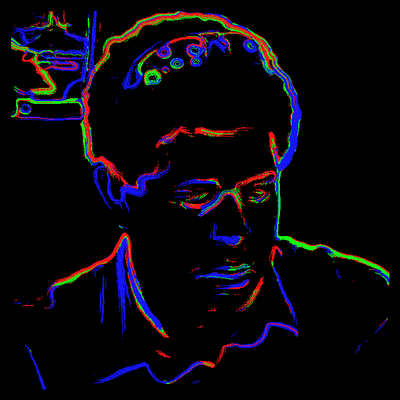Jose Ramirez Heran, (move over the picture to see the original, click for larger)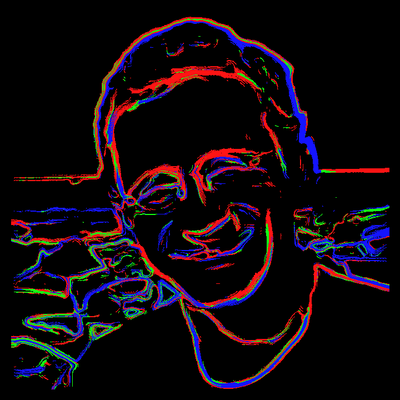Oliver Knill, (move over the picture to see the original, click for larger)